Welcome back, friend.

In the last two posts, I described the basics of how to build and manipulate the Fibonacci anyon vector space in Haskell.

As a personal anecdote, trying to understand the category theory behind the theory of anyons is one of the reasons I started learning Haskell. These spaces are typically described using the terminology of category theory. I found it very frustrating that anyons were described in an abstract and confusing terminology. I really wondered if people were just making things harder than they have to be. I think Haskell is a perfect playground to clarify these constructions. While the category theory stuff isn’t strictly necessary, it is interesting and useful once you get past the frustration.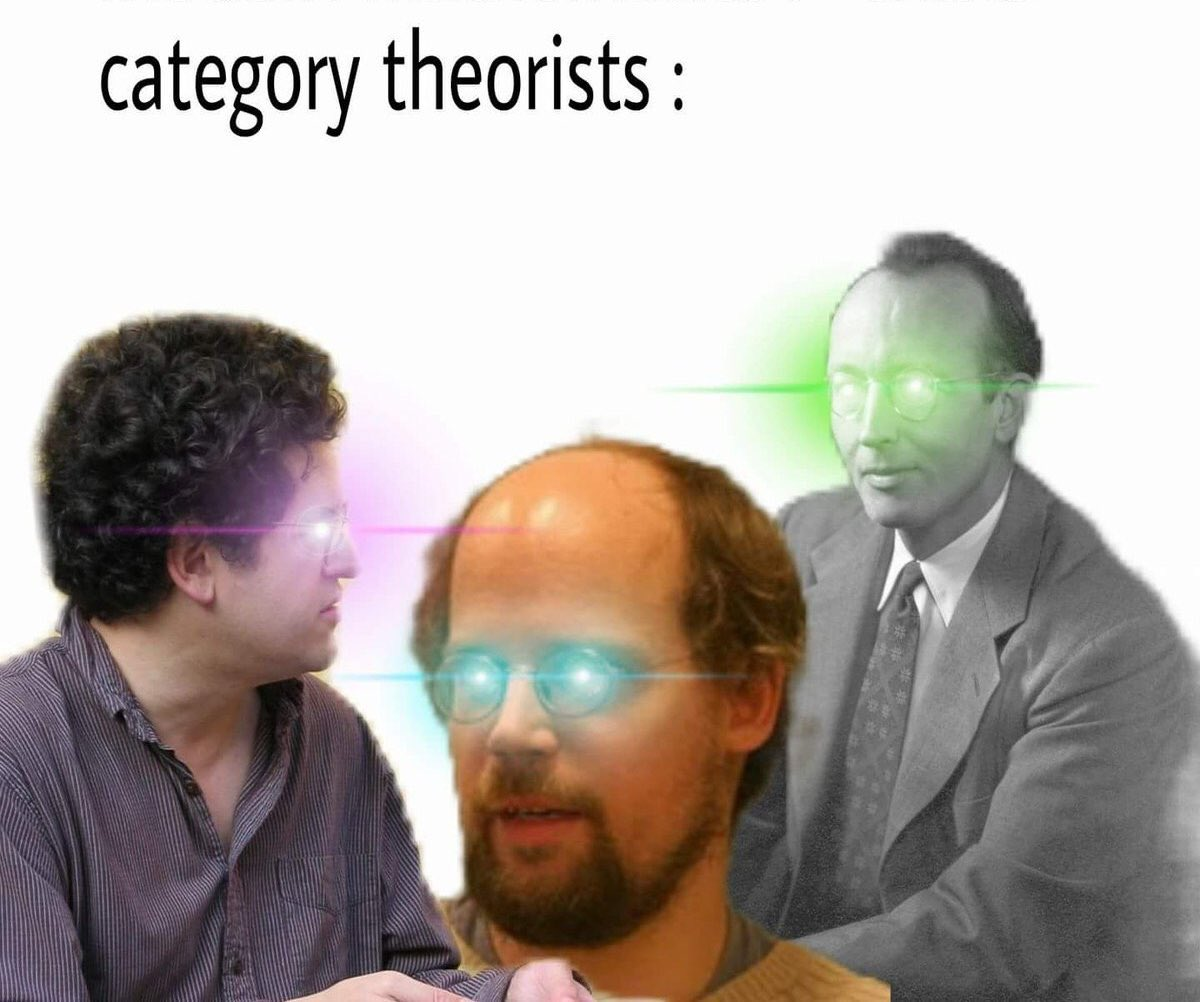but I hope everyone can get something out of it. Give it a shot if you’re interested, and don’t sweat the details.

#### The Aroma of Categories

I think Steve Awodey gives an excellent nutshell of category theory in the introductory section to his book:

“What is category theory? As a first approximation, one could say that category theory is the mathematical study of (abstract) algebras of functions. Just as group theory is the abstraction of the idea of a system of permutations of a set or symmetries of a geometric object, so category theory arises from the idea of a system of functions among some objects.”

For my intuition, a category is any “things” that plug together. The “in” of a thing has to match the “out” of another thing in order to hook them together. In other words, the requirement for something to be a category is having a notion of composition. The things you plug together are called the morphisms of the category and the matching ports are the objects of the category. The additional requirement of always having an identity morphism (a do-nothing connection wire) is usually there once you have composition, although it is good to take especial note of it.

Category theory is an elegant framework for how to think about these composing things in a mathematical way. In my experience, thinking in these terms leads to good abstractions, and useful analogies between disparate things.

It is helpful for any abstract concept to list some examples to expose the threads that connect them. Category theory in particular has a ton of examples connecting to many other fields because it is a science of analogy. These are the examples of categories I usually reach for. Which one feels the most comfortable to you will depend on your background.

• Hask. Objects are types. Morphisms are functions between those types
• Vect. Objects are vector spaces, morphisms are linear maps (roughly matrices).
• Preorders. Objects are values. Morphisms are the inequalities between those values.
• Sets. Objects are Sets. Morphisms are functions between sets.
• Cat. Objects are categories, Morphisms are functors. This is a pretty cool one, although complete categorical narcissism.
• Systems and Processes.
• The Free Category of a directed graphs. Objects are vertices. Morphisms are paths between vertices

#### Generic Programming and Typeclasses

The goal of generic programming is to run programs that you write once in many way.

There are many ways to approach this generic programming goal, but one way this is achieved in Haskell is by using Typeclasses. Typeclasses allow you to overload names, so that they mean different things based upon the types involved. Adding a vector is different than adding a float or int, but there are programs that can be written that reasonably apply in both situations.

Writing your program in a way that it applies to disparate objects requires abstract ways of talking about things. Mathematics is an excellent place to mine for good abstractions. In particular, the category theory abstraction has demonstrated itself to be a very useful unified vocabulary for mathematical topics. I, and others, find it also to be a beautiful aesthetic by which to structure programs.

In the Haskell base library there is a Category typeclass defined in base. In order to use this, you need to import the Prelude in an unusual way.


{-# LANGUAGE NoImplicitPrelude #-}
import Prelude hiding ((.), id)


The Category typeclass is defined on the type that corresponds to the morphisms of the category. This type has a slot for the input type and a slot for the output type. In order for something to be a category, it has to have an identity morphisms and a notion of composition.


class Category cat where
id :: cat a a
(.) :: cat b c -> cat a b -> cat a c


The most obvious example of this Category typeclass is the instance for the ordinary Haskell function (->). The identity corresponds to the standard Haskell identity function, and composition to ordinary Haskell function composition.


instance Category (->) where
id = \x -> x
f . g = \x -> f (g x)


Another example of a category that we’ve already encountered is that of linear operators which we’ll call LinOp. LinOp is an example of a Kliesli arrow, a category built using monadic composition rather than regular function composition. In this case, the monad Q from my first post takes care of the linear pipework that happens between every application of a LinOp. The fish <=< operator is monadic composition from Control.Monad.


newtype LinOp a b = LinOp {runLin :: a -> Q b}
instance Category LinOp where
id = LinOp pure
(LinOp f) . (LinOp g) = LinOp (f <=< g)


A related category is the FibOp category. This is the category of operations on Fibonacci anyons, which are also linear operations. It is LinOp specialized to the Fibonacci anyon space. All the operations we’ve previously discussed (F-moves, braiding) are in this category.


newtype FibOp a b = FibOp {runFib :: (forall c. FibTree c a -> Q (FibTree c b))}
instance Category (FibOp) where
id = FibOp pure
(FibOp f) . (FibOp g) = FibOp (f <=< g)


The “feel” of category theory takes focus away from the objects and tries to place focus on the morphisms. There is a style of functional programming called “point-free” where you avoid ever giving variables explicit names and instead use pipe-work combinators like (.), fst, snd, or (***). This also has a feel of de-emphasizing objects. Many of the combinators that get used in this style have categorical analogs. In order to generically use categorical typeclasses, you have to write your program in this point free style.

It is possible for a program written in the categorical style to be a reinterpreted as a program, a linear algebra operation, a circuit, or a diagram, all without changing the actual text of the program. For more on this, I highly recommend Conal Elliot’s compiling to categories, which also puts forth a methodology to avoid the somewhat unpleasant point-free style using a compiler plug-in. This might be an interesting place to mine for a good quantum programming language. YMMV.

### Monoidal Categories.

Putting two processes in parallel can be considered a kind of product. A category is monoidal )if it has this product of this flavor, and has isomorphisms for reassociating objects and producing or consuming a unit object. This will make more sense when you see the examples.

We can sketch out this monoidal category concept as a typeclass, where we use () as the unit object.


class Category k => Monoidal k where
parC :: k a c -> k b d -> k (a,b) (c,d)
assoc :: k ((a,b),c) (a,(b,c))
assoc' :: k (a,(b,c)) ((a,b),c)
leftUnitor :: k ((),a) a
leftUnitor' :: k a ((),a)
rightUnitor :: k (a,()) a
rightUnitor' :: k a (a,())


#### Instances

In Haskell, the standard monoidal product for regular Haskell functions is (***) from Control.Arrow. It takes two functions and turns it into a function that does the same stuff, but on a tuple of the original inputs. The associators and unitors are fairly straightforward. We can freely dump unit () and get it back because there is only one possible value for it.


(***) :: (a -> c) -> (b -> d) -> ((a,b) -> (c,d))
f *** g = \(x,y) -> (f x, g y) ﻿


instance Monoidal (->) where
parC f g = f *** g
assoc ((x,y),z) = (x,(y,z))
assoc' (x,(y,z)) = ((x,y),z)
leftUnitor (_, x) = x
leftUnitor' x = ((),x)
rightUnitor (x, _) = x
rightUnitor' x = (x,())


The monoidal product we’ll choose for LinOp is the tensor/outer/Kronecker product.


kron :: Num b => W b a -> W b c -> W b (a,c)
kron (W x) (W y) = W [((a,c), r1 * r2) | (a,r1) <- x , (c,r2) <- y ]


Otherwise, LinOp is basically a monadically lifted version of (->). The one dimensional vector space Q () is completely isomorphic to just a number. Taking the Kronecker product with it is basically the same thing as scalar multiplying (up to some shuffling).


instance Monoidal LinOp where
parC (LinOp f) (LinOp g) = LinOp $\(a,b) -> kron (f a) (g b) assoc = LinOp (pure . assoc) assoc' = LinOp (pure . unassoc) leftUnitor = LinOp (pure . leftUnitor) leftUnitor' = LinOp (pure .leftUnitor') rightUnitor = LinOp (pure . rightUnitor) rightUnitor' = LinOp (pure . rightUnitor')  Now for a confession. I made a misstep in my first post. In order to make our Fibonacci anyons jive nicely with our current definitions, I should have defined our identity particle using type Id = () rather than data Id. We’ll do that now. In addition, we need some new primitive operations for absorbing and emitting identity particles that did not feel relevant at that time.  rightUnit :: FibTree e (a,Id) -> Q (FibTree e a) rightUnit (TTI t _) = pure t rightUnit (III t _) = pure t rightUnit' :: FibTree e a -> Q (FibTree e (a,Id)) rightUnit' t@(TTT _ _) = pure (TTI t ILeaf) rightUnit' t@(TTI _ _) = pure (TTI t ILeaf) rightUnit' t@(TIT _ _) = pure (TTI t ILeaf) rightUnit' t@(III _ _) = pure (III t ILeaf) rightUnit' t@(ITT _ _) = pure (III t ILeaf) rightUnit' t@(ILeaf) = pure (III t ILeaf) rightUnit' t@(TLeaf) = pure (TTI t ILeaf) leftUnit :: FibTree e (Id,a) -> Q (FibTree e a) leftUnit = rightUnit <=< braid -- braid vs braid' doesn't matter, but it has a nice symettry. leftUnit' :: FibTree e a -> Q (FibTree e (Id,a)) leftUnit' = braid' <=< rightUnit'  With these in place, we can define a monoidal instance for FibOp. The extremely important and intriguing F-move operations are the assoc operators for the category. While other categories have assoc that feel nearly trivial, these F-moves don’t feel so trivial.  instance Monoidal (FibOp) where parC (FibOp f) (FibOp g) = (FibOp (lmap f)) . (FibOp (rmap g)) assoc = FibOp fmove' assoc' = FibOp fmove leftUnitor = FibOp leftUnit leftUnitor' = FibOp leftUnit' rightUnitor = FibOp rightUnit rightUnitor' = FibOp rightUnit'  #### This is actually useful The parC operation is extremely useful to explicitly note in a program. It is an opportunity for optimization. It is possible to inefficiently implement parC in terms of other primitives, but it is very worthwhile to implement it in new primitives (although I haven’t here). In the case of (->), parC is an explicit location where actual computational parallelism is available. Once you perform parC, it is not longer obviously apparent that the left and right side of the tuple share no data during the computation. In the case of LinOp and FibOp, parC is a location where you can perform factored linear computations. The matrix vector product$ (A \otimes B)(v \otimes w)$can be performed individually$ (Av)\otimes (Bw)$. In the first case, where we densify$ A \otimes B$and then perform the multiplication, it costs$ O((N_A N_B)^2)$time, whereas performing them individually on the factors costs$ O( N_A^2 + N_B^2)$time, a significant savings. Applied category theory indeed. #### Laws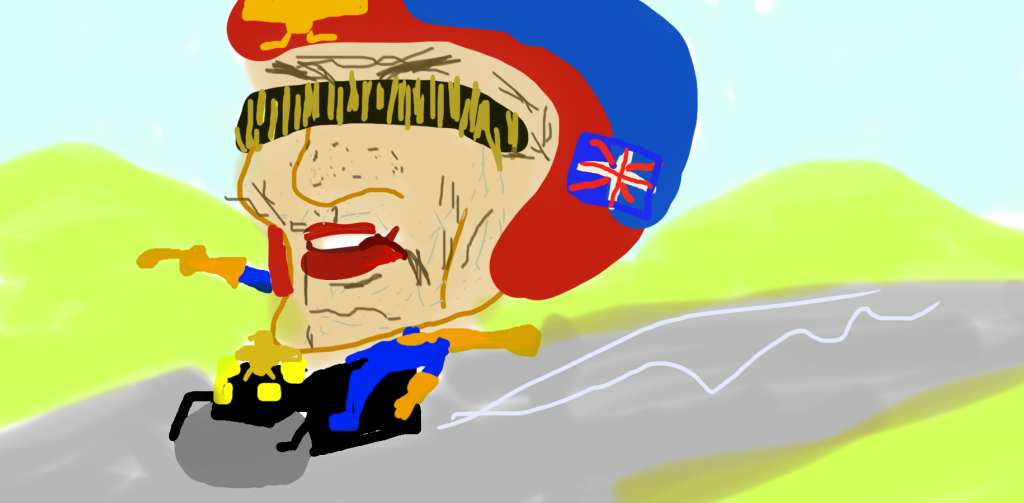Judge Dredd courtesy of David Like many typeclasses, these monoidal morphisms are assumed to follow certain laws. Here is a sketch (for a more thorough discussion check out the wikipedia page): • Functions with a tick at the end like assoc' should be the inverses of the functions without the tick like assoc, e.g. assoc . assoc' = id • The parC operation is (bi)functorial, meaning it obeys the commutation law parC (f . f') (g . g') = (parC f g) . (parC f' g') i.e. it doesn’t matter if we perform composition before or after the parC. • The pentagon law for assoc: Applying leftbottom is the same as applying toprightleftbottom :: (((a,b),c),d) -> (a,(b,(c,d))) leftbottom = assoc . assoc topright :: (((a,b),c),d) -> (a,(b,(c,d))) topright = (id *** assoc) . assoc . (assoc *** id)  • The triangle law for the unitors:topright' :: ((a,()),b) -> (a,b) topright' = (id *** leftUnitor) . assoc leftside :: ((a,()),b) -> (a,b) leftside = rightUnitor *** id  #### String Diagrams String diagrams are a diagrammatic notation for monoidal categories. Morphisms are represented by boxes with lines.Composition g . f is made by connecting lines.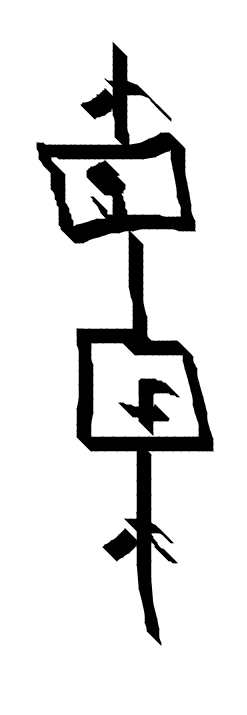The identity id is a raw arrow.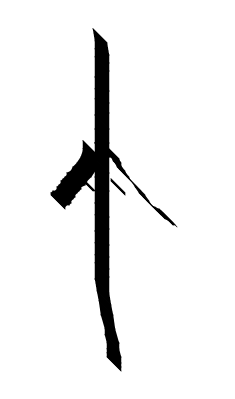The monoidal product of morphisms$ f \otimes g$is represented by placing lines next to each other.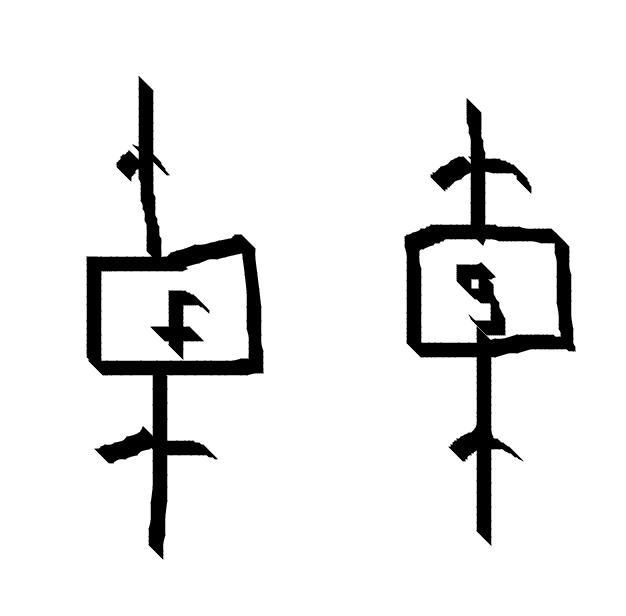The diagrammatic notion is so powerful because the laws of monoidal categories are built so deeply into it they can go unnoticed. Identities can be put in or taken away. Association doesn’t even appear in the diagram. The boxes in the notation can naturally be pushed around and commuted past each other.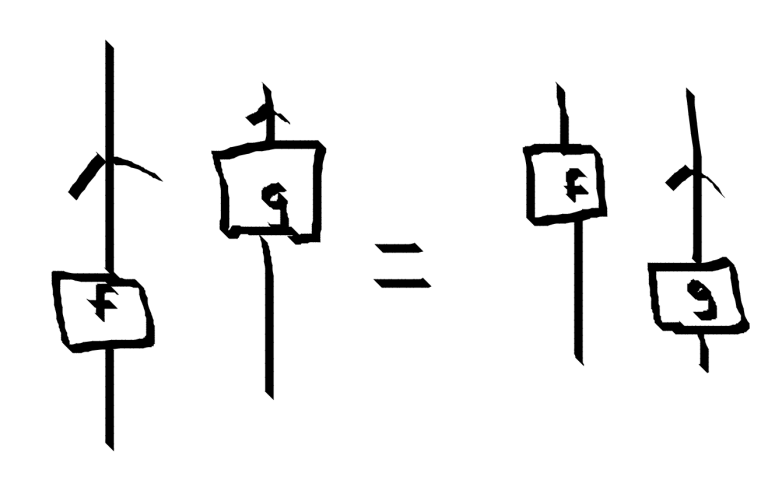This corresponds to the property$ (id \otimes g) \circ (f \otimes id) = (f \otimes id) \circ (id \otimes g)$What expression does the following diagram represent?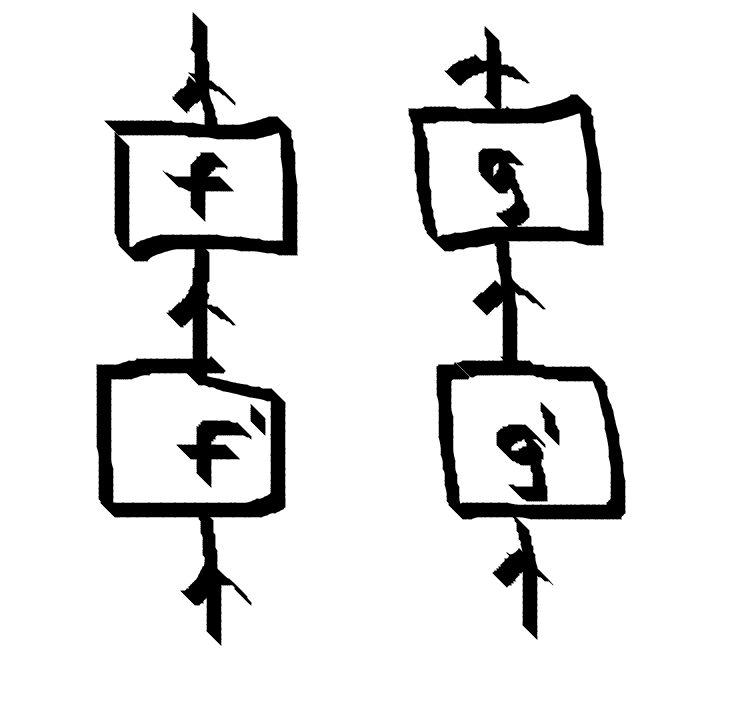Is it$ (f \circ f’) \otimes (g \circ g’)$(in Haskell notation parC (f . f') (g . g') )? Or is it$ (f \otimes g) \circ (f’ \otimes g’)$(in Haskell notation (parC f g) . (parC f' g')? Answer: It doesn’t matter because the functorial requirement of parC means the two expressions are identical. There are a number of notations you might meet in the world that can be interpreted as String diagrams. Three that seem particular pertinent are: • Quantum circuits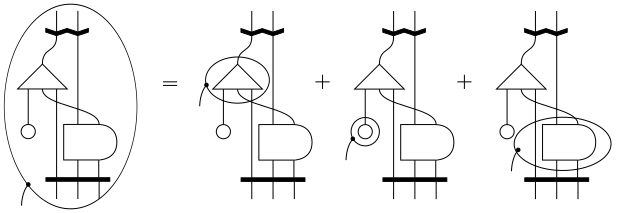• Anyon Diagrams!#### Braided and Symmetric Monoidal Categories: Categories That Braid and Swap Some monoidal categories have a notion of being able to braid morphisms. If so, it is called a braided monoidal category (go figure).  class Monoidal k => Braided k where over :: k (a,b) (b,a) under :: k (a,b) (b,a)  The over and under morphisms are inverse of each other over . under = id. The over morphism pulls the left morphism over the right, whereas the under pulls the left under the right. The diagram definitely helps to understand this definition.These over and under morphisms need to play nice with the associator of the monoidal category. These are laws that valid instance of the typeclass should follow. We actually already met them in the very first post. If the over and under of the braiding are the same the category is a symmetric monoidal category. This typeclass needs no extra functions, but it is now intended that the law over . over = id is obeyed.  class Braided k => Symmetric k where  When we draw a braid in a symmetric monoidal category, we don’t have to be careful with which one is over and under, because they are the same thing.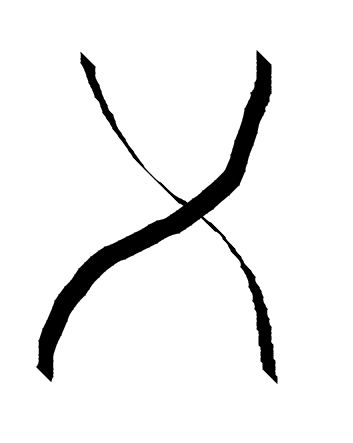The examples that come soonest to mind have this symmetric property, for example (->) is a symmetric monoidal category..  swap :: (a, b) -> (b, a) swap (x,y) = (y,x)   instance Braided (->) where over = swap under = swap instance Symmetric (->)  Similarly LinOp has an notion of swapping that is just a lifting of swap  instance Braided (LinOp) where over = LinOp (pure . swap) under = LinOp (pure . swap) instance Symmetric LinOp  However, FibOp is not symmetric! This is perhaps at the core of what makes FibOp so interesting.  instance Braided FibOp where over = FibOp braid under = FibOp braid'  #### Automating Association Last time, we spent a lot of time doing weird typelevel programming to automate the pain of manual association moves. We can do something quite similar to make the categorical reassociation less painful, and more like the carefree ideal of the string diagram if we replace composition (.) with a slightly different operator  (...) :: ReAssoc b b' => FibOp b' c -> FibOp a b -> FibOp a c (FibOp f) ... (FibOp g) = FibOp$ f <=< reassoc <=< g


Before defining reassoc, let’s define a helper LeftCollect typeclass. Given a typelevel integer n, it will reassociate the tree using a binary search procedure to make sure the left branch l at the root has Count l = n.


leftcollect :: forall n gte l r o e. (gte ~ CmpNat n (Count l), LeftCollect n gte (l,r) o) => FibTree e (l,r) -> Q (FibTree e o)
leftcollect x = leftcollect' @n @gte x

class LeftCollect n gte a b | n gte a -> b where
leftcollect' :: FibTree e a -> Q (FibTree e b)

-- The process is like a binary search.
-- LeftCollect pulls n leaves into the left branch of the tuple

-- If n is greater than the size of l, we recurse into the right branch with a new number of leaves to collect
-- then we do a final reshuffle to put those all into the left tree.
instance (
k ~ Count l,
r ~ (l',r'),
n' ~ (n - k),
gte ~ CmpNat n' (Count l'),
LeftCollect n' gte r (l'',r'')) => LeftCollect n 'GT (l,r) ((l,l''),r'') where
leftcollect' x = do
x' <- rmap (leftcollect @n') x -- (l,(l'',r'')) -- l'' is size n - k
fmove x'  -- ((l,l''),r'') -- size of (l,l'') = k + (n-k) = n
instance (
l ~ (l',r'),
gte ~ CmpNat n (Count l'),
LeftCollect n gte l (l'',r'')) => LeftCollect n 'LT (l,r) (l'',(r'',r)) where
leftcollect' x = do
x' <- lmap (leftcollect @n) x -- ((l'',r''),r) -- l'' is of size n
fmove' x'  -- (l'',(r'',r)

instance LeftCollect n 'EQ (l,r) (l,r) where
leftcollect' = pure


Once we have LeftCollect, the typeclass ReAssoc is relatively simple to define. Given a pattern tree, we can count the elements in it’s left branch and LeftCollect the source tree to match that number. Then we recursively apply reassoc in the left and right branch of the tree. This means that every node has the same number of children in the tree, hence the trees will end up in an identical shape (modulo me mucking something up).


class ReAssoc a b where
reassoc :: FibTree e a -> Q (FibTree e b)
instance (n ~ Count l',
gte ~ CmpNat n (Count l),
LeftCollect n gte (l,r) (l'',r''),
ReAssoc l'' l',
ReAssoc r'' r') => ReAssoc (l,r) (l',r') where
reassoc x = do
x' <- leftcollect @n x
x'' <- rmap reassoc x'
lmap reassoc x''

--instance {-# OVERLAPS #-} ReAssoc a a where
--   reassoc = pure

instance ReAssoc Tau Tau where
reassoc = pure
instance ReAssoc Id Id where
reassoc = pure


It seems likely that one could write equivalent instances that would work for an arbitrary monoidal category with a bit more work. We are aided somewhat by the fact that FibOp has a finite universe of possible leaf types to work with.

### Closing Thoughts

While our categorical typeclasses are helpful and nice, I should point out that they are not going to cover all the things that can be described as categories, even in Haskell. Just like the Functor typeclass does not describe all the conceptual functors you might meet. One beautiful monoidal category is that of Haskell Functors under the monoidal product of Functor Composition. More on this to come, I think. https://parametricity.com/posts/2015-07-18-braids.html

We never even touched the dot product in this post. This corresponds to another doodle in a string diagram, and another power to add to your category. It is somewhat trickier to work with cleanly in familiar Haskell terms, I think because (->) is at least not super obviously a dagger category?

You can find a hopefully compiling version of all my snippets and more in my chaotic mutating Github repo https://github.com/philzook58/fib-anyon

See you next time.

The Rosetta Stone paper by Baez and Stay is probably the conceptual daddy of this entire post (and more).

Bartosz Milewski’s Category Theory for Programmer’s blog (online book really) and youtube series are where I learned most of what I know about category theory. I highly recommend them (huge Bartosz fanboy).

https://www.math3ma.com/blog/what-is-category-theory-anyway

There are fancier embeddings of category theory and monoidal categories than I’ve shown here. Often you want constrained categories and the ability to choose unit objects. I took a rather simplistic approach here.